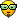# reading grove sensor data on a grove xbee carrier and rn171

hi
i have read a post on wifly pins but after a lot of reading and search, i dont have some clear ideasi have 3 grove bee carrier with a rn xv 171 and grove sensors (light, barometer, temperature…)

i receive the data by a GET /myscipt.php?DATA= XXXX00001111222233334444555566667777\n\n
Where XXXX is the GPIO data and 0000 through to 7777 are the values of the 8 analogue inputs expressed in hexadecimal.

my problem is that i dont understand to which pins are output data on grove carrier and the corresponding channel sensors on The rnXV-I/RM . there is 2 connectors on the grove xbee carrier and some sensor have 2 functions like the dth11 (humidity and temperature). how can i know that for example the d6 and the d5 wire connector or scl or sda on the xbee carrier are link on which channel on the wifly.

For exemple,i have the calculation for the light sensor based on the millivolt data but i dont know to search in the get data process because each channel with the show q command give a result
For dth11 with humidity and temperature i dont have the algorithm and i dont know what channel are involved when i put in d5 d6 connectors.each channel with the show q command give a result
For the barometric sensor, there are 4 results pressure, altitude, atmosphere and tempeature. i see in the datasheet some calculation but in this case, i dont know which data to use on the 8 channels results

thanks for a lighting idea on the pin data order on the grove carrier and a the sensors channels which can permit to have the data on my php script for doing the calculation. :doh:

thanks and forgive me for bad english
bruno

about some readings, it seems that sen5 is link to grove d5d6 connector and sen2 to the scl sda connector

I read but dont understand how to have a precision reference voltage on one of the analog
inputs to calculate the offset.
Input voltage range: 0-1.2V, however, the analog-to-digital converter saturates at 400 mV
Resolution: 14 bits = 12 uV
Sampling frequency: 35 us
WARNING
Driving these inputs above 1.2V can permanently damage the module.
show q 0x183 // Read channels 0, 1, and 7
Accuracy: 5% uncalibrated
The accuracy of each analog sensor reading can be offset by up to 5% due to variations
from chip to chip. To improve accuracy, Microchip recommends using a precision reference voltage on one of the analog
inputs to calculate the offset.

If you read 210 mV you know that the offset is +10 mV. When you read input 5, subtract 10 mV from the result.

And another thing, i use dth11 grove sensor. this sensor use a 40 bytes data to give humidity and temperature. i use channel 5 by a http get request with a php script.

i receive the data by a GET /myscipt.php?DATA= XXXX00001111222233334444555566667777\n\n
Where XXXX is the GPIO data and 0000 through to 7777 are the values of the 8 analogue inputs expressed in hexadecimal.

this is the way dth11 data are done. the 7777 values arent too small to content the 40bytes of the dth 11 data i’m not a mathematic convesion man… ? in that case how can i tansmit the 40bytes of data

DATA=16 bits RH data+16 bits Temperature data+8 bits check-sum
Example: MCU has received 40 bits data from RHT03 as
0000 0010 1000 1100 0000 0001 0101 1111 1110 1110
16 bits RH data 16 bits T data check sum
Here we convert 16 bits RH data from binary system to decimal system,
0000 0010 1000 1100 → 652
Binary system Decimal system
RH=652/10=65.2%RH
Here we convert 16 bits T data from binary system to decimal system,
0000 0001 0101 1111 → 351
Binary system Decimal system
T=351/10=35.1℃
When highest bit of temperature is 1, it means the temperature is below 0 degree Celsius.
Example: 1000 0000 0110 0101, T= minus 10.1℃
16 bits T data
Sum=0000 0010+1000 1100+0000 0001+0101 1111=1110 1110
Check-sum=the last 8 bits of Sum=1110 1110

thanks